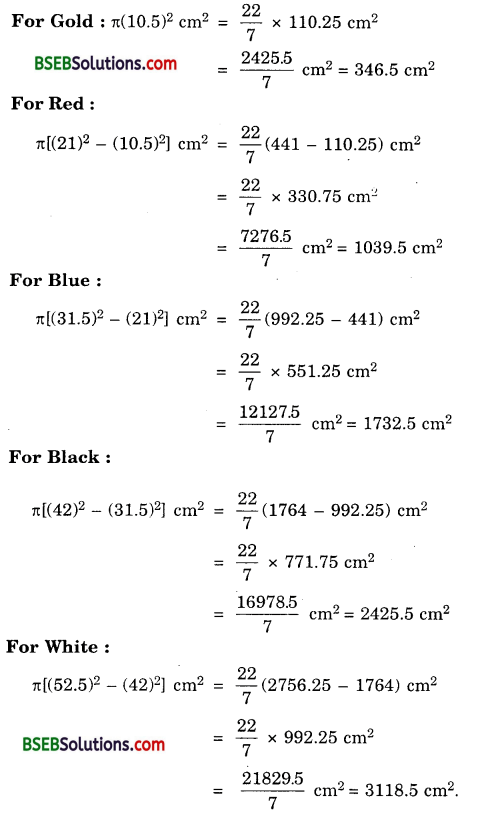# Bihar Board Class 10th Maths Solutions Chapter 12 Areas Related to Circles Ex 12.1

Bihar Board Class 10th Maths Solutions Chapter 12 Areas Related to Circles Ex 12.1 Textbook Questions and Answers.

## BSEB Bihar Board Class 10th Maths Solutions Chapter 12 Areas Related to Circles Ex 12.1Question 1.
The radii of two circles are 19 cm and 9 cm respectively. Find the radius of the circle which has circumference equal to the sum of the circumferences of the two circles.
Solution:
Let r be the radius of the circle whose circumference is equal to the sum of the circumferences of the two circles of radii 19 cm and 9 cm.
∴ 2πr = 2π( 19) + 2π(9)
or r = 19 + 9 = 28
Hence, the radius of the new circle is 28 cm.

Question 2.
The radii of two circles are 8 cm and 6 cm respectively. Find the radius of the circle having area equal to the sum of the areas of the two circles.
Solution:
Let r be the radius of the circle whose area is equal to the sum of the areas of the circles of radii 8 cm and 6 cm.
∴ π² = π(8)² + π(6)² or r² = 8² + 6²
or r² = 64 + 36 or r² = 100
or r = $$\sqrt{100}$$ = 10
Hence, the radius of the new circle is 10 cm.Question 3.
Figure depicts an archery target marked with its five scoring areas from the centre outwards as Gold, Red, Blue, Black and White. The diameter of the region representing Gold score is 21 cm and each of the other bands is 10.5 cm wide. Find the area of each of the five scoring regions.Solution:
The area of each of five scoring regions are as under :Question 4.
The wheels of a car are of diameter 80 cm each. How many complete revolutions does each wheel make in 10 minutes when the car is travelling at a speed of 66 km per hour?
Solution:
Distance covered by the car in 10 minutes
= $$\left(\frac{66 \times 1000 \times 100 \times 10}{60}\right)$$ cm
= $$\frac{6600000}{6}$$ = 1100000 cm
Circumference of each wheel of the car
= 2πr = $$\left(2 \times \frac{22}{7} \times 40\right)$$cm [∴ r = $$\frac{80}{2}$$ cm = 40]
Number of revolutions in 10 minutes when the car is travelling at a speed of 66 km/h = 1100000 ÷ (2 x $$\frac{22}{5}$$ x 40)
= 4375
Hence, each wheel of car makes 4375 revolutions in 10 minutes.Question 5.
Tick the correct answer in the following and justify your choice : If the perimeter and the area of a circle are numerically equal, then the radius of the circle is
(A) 2 units
(B) it units
(C) 4 units
(D) 7 units
Solution:
(A) Because,
Here, 2πr = πr², where r is the radius.
So, r² – 2r = 0 or r(r – 2) = 0
or r = 0 or r = 2
But, r ≠ 0. r = 2 units